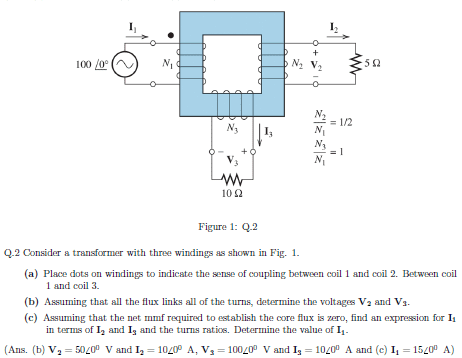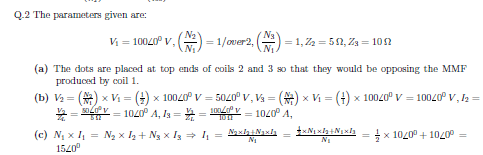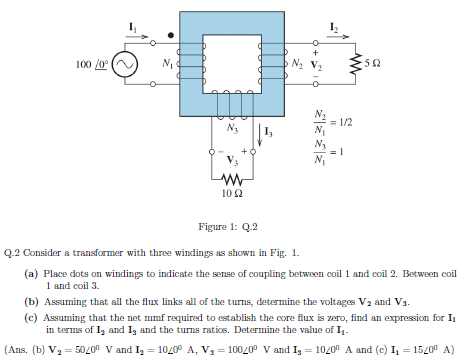# Transformer dot prob don't understand (with sol)

The problem:Solution in the spoiler:I don't know how to do the problem (a), but I also don't understand the answer (top of coil 3?). Can you give me the process on how to do the problem (a), i.e. in general how do I assign dots to coils in circuits? :)

#### Attachments

The problem:Solution in the spoiler:I don't know how to do the problem (a), but I also don't understand the answer (top of coil 3?). Can you give me the process on how to do the problem (a), i.e. in general how do I assign dots to coils in circuits? :)

the answer was wrong, it should be right side for coil 3, after finding that out i understand.

Assume that the conductor enters the core from the back and exits the core from the front.
Put a dot where the conductor exits the core.
On the left hand coil the conductor exits the core on the top. (It enters the core on the bottom.
On the right hand coil the conductor exits the core on the top. Put a dot at the top of the right hand coil.
On the bottom coil the conductor exits the core on the right. Put a dot to the right of the bottom coil.